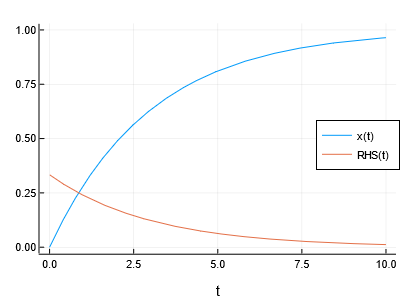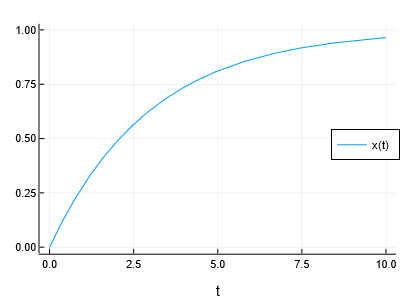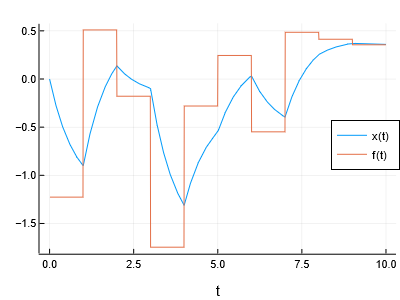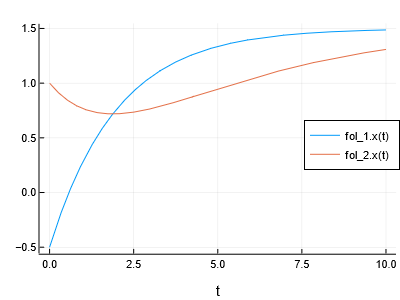# Composing Ordinary Differential Equations

This is an introductory example for the usage of ModelingToolkit (MTK). It illustrates the basic user-facing functionality by means of some examples of Ordinary Differential Equations (ODE). Some references to more specific documentation are given at appropriate places.

## Copy-Pastable Simplified Example

A much deeper tutorial with forcing functions and sparse Jacobians is all below. But if you want to just see some code and run, here's an example:

using ModelingToolkit

@variables t x(t) RHS(t)  # independent and dependent variables
@parameters τ       # parameters
D = Differential(t) # define an operator for the differentiation w.r.t. time

# your first ODE, consisting of a single equation, indicated by ~
@named fol_separate = ODESystem([ RHS  ~ (1 - x)/τ,
D(x) ~ RHS ])

using DifferentialEquations: solve
using Plots: plot

prob = ODEProblem(structural_simplify(fol_separate), [x => 0.0], (0.0,10.0), [τ => 3.0])
sol = solve(prob)
plot(sol, vars=[x,RHS])Now let's start digging into MTK!

Let us start with a minimal example. The system to be modelled is a

first-order lag element:

$$$\dot{x} = \frac{f(t) - x(t)}{\tau}$$$

Here, $t$ is the independent variable (time), $x(t)$ is the (scalar) state variable, $f(t)$ is an external forcing function, and $\tau$ is a constant parameter. In MTK, this system can be modelled as follows. For simplicity, we first set the forcing function to a constant value.

using ModelingToolkit

@variables t x(t)  # independent and dependent variables
@parameters τ       # parameters
D = Differential(t) # define an operator for the differentiation w.r.t. time

# your first ODE, consisting of a single equation, indicated by ~
@named fol_model = ODESystem(D(x) ~ (1 - x)/τ)
# Model fol_model with 1 equations
# States (1):
#   x(t)
# Parameters (1):
#   τ

Note that equations in MTK use the tilde character (~) as equality sign. Also note that the @named macro simply ensures that the symbolic name matches the name in the REPL. If omitted, you can directly set the name keyword.

After construction of the ODE, you can solve it using DifferentialEquations.jl:

using DifferentialEquations
using Plots

prob = ODEProblem(fol_model, [x => 0.0], (0.0,10.0), [τ => 3.0])
plot(solve(prob))The initial state and the parameter values are specified using a mapping from the actual symbolic elements to their values, represented as an array of Pairs, which are constructed using the => operator.

## Algebraic relations and structural simplification

You could separate the calculation of the right-hand side, by introducing an intermediate variable RHS:

@variables RHS(t)
@named fol_separate = ODESystem([ RHS  ~ (1 - x)/τ,
D(x) ~ RHS ])
# Model fol_separate with 2 equations
# States (2):
#   x(t)
#   RHS(t)
# Parameters (1):
#   τ

To directly solve this system, you would have to create a Differential-Algebraic Equation (DAE) problem, since besides the differential equation, there is an additional algebraic equation now. However, this DAE system can obviously be transformed into the single ODE we used in the first example above. MTK achieves this by means of structural simplification:

fol_simplified = structural_simplify(fol_separate)

equations(fol_simplified)
# 1-element Array{Equation,1}:
#  Differential(t)(x(t)) ~ (τ^-1)*(1 - x(t))

equations(fol_simplified) == equations(fol_model)
# true

You can extract the equations from a system using equations (and, in the same way, states and parameters). The simplified equation is exactly the same as the original one, so the simulation performance will also be the same. However, there is one difference. MTK does keep track of the eliminated algebraic variables as "observables" (see Observables and Variable Elimination). That means, MTK still knows how to calculate them out of the information available in a simulation result. The intermediate variable RHS therefore can be plotted along with the state variable. Note that this has to be requested explicitly, though:

prob = ODEProblem(fol_simplified, [x => 0.0], (0.0,10.0), [τ => 3.0])
sol = solve(prob)
plot(sol, vars=[x, RHS])Note that similarly the indexing of the solution works via the names, and so sol[x] gives the timeseries for x, sol[x,2:10] gives the 2nd through 10th values of x matching sol.t, etc. Note that this works even for variables which have been eliminated, and thus sol[RHS] retrieves the values of RHS.

## Specifying a time-variable forcing function

What if the forcing function (the "external input") $f(t)$ is not constant? Obviously, one could use an explicit, symbolic function of time:

@variables f(t)
@named fol_variable_f = ODESystem([f ~ sin(t), D(x) ~ (f - x)/τ])

But often there is time-series data, such as measurement data from an experiment, we want to embed as data in the simulation of a PDE, or as a forcing function on the right-hand side of an ODE – is it is the case here. For this, MTK allows to "register" arbitrary Julia functions, which are excluded from symbolic transformations but are just used as-is. So, you could, for example, interpolate a given time series using DataInterpolations.jl. Here, we illustrate this option by a simple lookup ("zero-order hold") of a vector of random values:

value_vector = randn(10)
f_fun(t) = t >= 10 ? value_vector[end] : value_vector[Int(floor(t))+1]
@register_symbolic f_fun(t)

@named fol_external_f = ODESystem([f ~ f_fun(t), D(x) ~ (f - x)/τ])
prob = ODEProblem(structural_simplify(fol_external_f), [x => 0.0], (0.0,10.0), [τ => 0.75])

sol = solve(prob)
plot(sol, vars=[x,f])## Building component-based, hierarchical models

Working with simple one-equation systems is already fun, but composing more complex systems from simple ones is even more fun. Best practice for such a "modeling framework" could be to use factory functions for model components:

function fol_factory(separate=false;name)
@parameters τ
@variables t x(t) f(t) RHS(t)

eqs = separate ? [RHS ~ (f - x)/τ,
D(x) ~ RHS] :
D(x) ~(f - x)/τ

ODESystem(eqs;name)
end

Such a factory can then used to instantiate the same component multiple times, but allows for customization:

@named fol_1 = fol_factory()
@named fol_2 = fol_factory(true) # has observable RHS

Now, these two components can be used as subsystems of a parent system, i.e. one level higher in the model hierarchy. The connections between the components again are just algebraic relations:

connections = [ fol_1.f ~ 1.5,
fol_2.f ~ fol_1.x ]

connected = compose(ODESystem(connections,name=:connected), fol_1, fol_2)
# Model connected with 5 equations
# States (5):
#   fol_1₊f(t)
#   fol_2₊f(t)
#   fol_1₊x(t)
#   fol_2₊x(t)
# ⋮
# Parameters (2):
#   fol_1₊τ
#   fol_2₊τ

All equations, variables and parameters are collected, but the structure of the hierarchical model is still preserved. That is, you can still get information about fol_1 by addressing it by connected.fol_1, or its parameter by connected.fol_1.τ. Before simulation, we again eliminate the algebraic variables and connection equations from the system using structural simplification:

connected_simp = structural_simplify(connected)
# Model connected with 2 equations
# States (2):
#   fol_1₊x(t)
#   fol_2₊x(t)
# Parameters (2):
#   fol_1₊τ
#   fol_2₊τ
# Incidence matrix:
#   [1, 1]  =  ×
#   [2, 1]  =  ×
#   [2, 2]  =  ×
#   [1, 3]  =  ×
#   [2, 4]  =  ×

equations(connected_simp)
# 2-element Array{Equation,1}:
#  Differential(t)(fol_1₊x(t)) ~ (fol_1₊τ^-1)*(1.5 - fol_1₊x(t))
#  Differential(t)(fol_2₊x(t)) ~ (fol_2₊τ^-1)*(fol_1₊x(t) - fol_2₊x(t))

As expected, only the two state-derivative equations remain, as if you had manually eliminated as many variables as possible from the equations. As mentioned above, the hierarchical structure is preserved though. So the initial state and the parameter values can be specified accordingly when building the ODEProblem:

u0 = [ fol_1.x => -0.5,
fol_2.x => 1.0 ]

p = [ fol_1.τ => 2.0,
fol_2.τ => 4.0 ]

prob = ODEProblem(connected_simp, u0, (0.0,10.0), p)
plot(solve(prob))More on this topic may be found in Composing Models and Building Reusable Components.

## Defaults

Often it is a good idea to specify reasonable values for the initial state and the parameters of a model component. Then, these do not have to be explicitly specified when constructing the ODEProblem.

function unitstep_fol_factory(;name)
@parameters τ
@variables t x(t)
ODESystem(D(x) ~ (1 - x)/τ; name, defaults=Dict(x=>0.0, τ=>1.0))
end

ODEProblem(unitstep_fol_factory(name=:fol),[],(0.0,5.0),[]) |> solve

Note that the defaults can be functions of the other variables, which is then resolved at the time of the problem construction. Of course, the factory function could accept additional arguments to optionally specify the initial state or parameter values, etc.

## Symbolic and sparse derivatives

One advantage of a symbolic toolkit is that derivatives can be calculated explicitly, and that the incidence matrix of partial derivatives (the "sparsity pattern") can also be explicitly derived. These two facts lead to a substantial speedup of all model calculations, e.g. when simulating a model over time using an ODE solver.

By default, analytical derivatives and sparse matrices, e.g. for the Jacobian, the matrix of first partial derivatives, are not used. Let's benchmark this (prob still is the problem using the connected_simp system above):

using BenchmarkTools

@btime solve($prob, Rodas4()); # 251.300 μs (873 allocations: 31.18 KiB) Now have MTK provide sparse, analytical derivatives to the solver. This has to be specified during the construction of the ODEProblem: prob_an = ODEProblem(connected_simp, u0, (0.0,10.0), p; jac=true, sparse=true) @btime solve($prob_an, Rodas4());
# 142.899 μs (1297 allocations: 83.96 KiB)

The speedup is significant. For this small dense model (3 of 4 entries are populated), using sparse matrices is counterproductive in terms of required memory allocations. For large, hierarchically built models, which tend to be sparse, speedup and the reduction of memory allocation can be expected to be substantial. In addition, these problem builders allow for automatic parallelism using the structural information. For more information, see the ODESystem page.

## Notes and pointers how to go on

Here are some notes that may be helpful during your initial steps with MTK:

• Sometimes, the symbolic engine within MTK is not able to correctly identify the independent variable (e.g. time) out of all variables. In such a case, you usually get an error that some variable(s) is "missing from variable map". In most cases, it is then sufficient to specify the independent variable as second argument to ODESystem, e.g. ODESystem(eqs, t).
• A completely macro-free usage of MTK is possible and is discussed in a separate tutorial. This is for package developers, since the macros are only essential for automatic symbolic naming for modelers.
• Vector-valued parameters and variables are possible. A cleaner, more consistent treatment of these is work in progress, though. Once finished, this introductory tutorial will also cover this feature.

Where to go next?

• Not sure how MTK relates to similar tools and packages? Read Comparison of ModelingToolkit vs Equation-Based Modeling Languages.
• Depending on what you want to do with MTK, have a look at some of the other Symbolic Modeling Tutorials.
• If you want to automatically convert an existing function to a symbolic representation, you might go through the ModelingToolkitize Tutorials.
• To learn more about the inner workings of MTK, consider the sections under Basics and System Types.